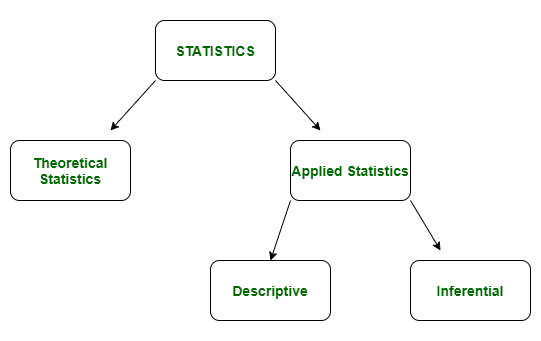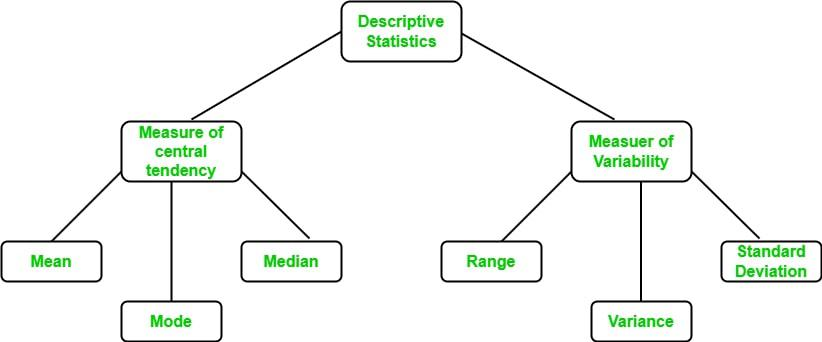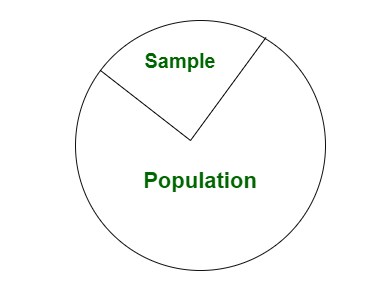# Difference between Descriptive and Inferential statistics

• Difficulty Level : Medium
• Last Updated : 31 Aug, 2022

Statistics is a branch of mathematics dealing with the collection, analysis, interpretation, and presentation of masses of numerical data. It is basically a collection of quantitative data.

Types of Statistics:

• Theoretical Statistics
• Applied Statistics1. Descriptive Statistics: Descriptive statistics is a term given to the analysis of data that helps to describe, show and summarize data in a meaningful way. It is a simple way to describe our data. Descriptive statistics is very important to present our raw data ineffective/meaningful way using numerical calculations or graphs or tables. This type of statistics is applied to already known data.

Types of Descriptive Statistics:

• Measure of Central Tendency
• Measure of Variability2. Inferential Statistics: In inferential statistics, predictions are made by taking any group of data in which you are interested. It can be defined as a random sample of data taken from a population to describe and make inferences about the population. Any group of data that includes all the data you are interested in is known as population. It basically allows you to make predictions by taking a small sample instead of working on the whole population.### Difference between Descriptive and Inferential statistics:

My Personal Notes arrow_drop_up